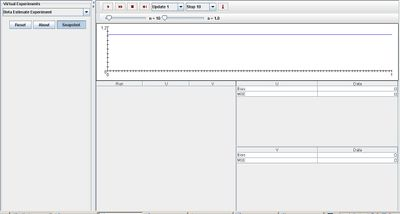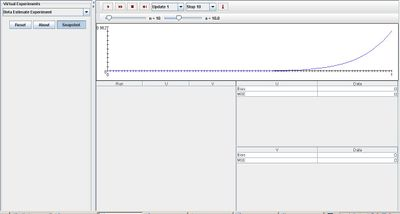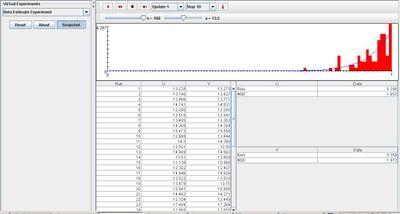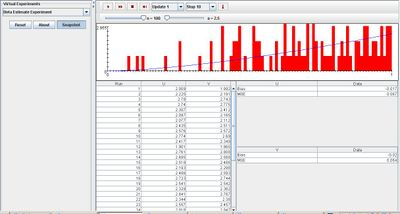# Difference between revisions of "SOCR EduMaterials Activities BetaEstimateExperiment"

## Description

Using the properties of Beta-distribution, the Beta Estimate Experiment illustrates the effects of bias and precision in parameter estimation.

The beta distribution consist of continuous probability distributions defined on an interval differing in the values of the two parameters, a and 1. This experiment is to generate a random sample X1, X2, ..., Xn of size n from the beta distribution.

On each update, the distribution density is shown in blue and the sample density is shown in red in the graph. Below the graph, the following information is recorded: U = M / (1-M) where M = (X1+X2+ ... +Xn) / n V = -n / [ln(X1)+ln(X2)+ ... +ln(Xn)] In the second table, the empirical bias and mean square error of each estimator are recorded as the experiment continues to run.

Statistics U and V are point estimators which are functions of the sample data that are used to estimate the unknown population parameter. It is referred to as an estimate of the actual application of the set of data.

The parameters a and n can be varied with scroll bars.

## Goal

To provide an accessible simulation to explore the function of beta distribution and its point estimators of a parameter. In order to estimate a parameter of interest, the point estimator of the parameter must be calculated from a random sample from the population. The variability is then calculated and associated with the parameter of interest.

## Experiment

Go to the SOCR Experiment [] and select the Beta Estimate Experiment from the drop-down list of experiments on the top left. The image below shows the initial view of this experiment:When pressing the play button, one trial will be executed and recorded in the distribution table below. The fast forward button symbolizes the nth number of trials to be executed each time. The stop button ceases any activity and is helpful when the experimenter chooses “continuous,” indicating an infinite number of events. The fourth button will reset the entire experiment, deleting all previous information and data collected. The “update” scroll indicates nth number of trials (1, 10, 100, or 1000) performed when selecting the fast forward button and the “stop” scroll indicates the maximum number of trials in the experiment.

The initial value of parameter a sets the density distribution graph in a linear horizontal fashion. When increasing parameter a, note that the density distribution graph becomes skewed left. The image below displays the effect of increasing value a:Since the value of n only increases the sample size, it does not greatly affect the outcome of the experiment. But varying parameter a influences the sample density graph shown in red. The experiment should realize that the larger the value of parameter a is, the more dense the sample density graph is. The image below displays an experiment with a large value of a after many trials:But when a does not obtain a large value, the sample density graph is has a large spread. The image below illustrates the experiment with a small value of a after many trials:## Applications

The Beta Estimate Experiment illustrates the bias and precision when sampling from a large population with a varying parameter. It could be useful in daily activities such as determining the behaviors of randomly selecting a student from a lecture hall. With the initial value of a, the experiment may represent an equal probability of selecting any student within the lecture hall, but with a large value of a, the experiment shows a bias in which students sitting in the first three rows may have a higher change of being selected.